### 2011 SSC CGL Tier 2 Quantitative Aptitude Question Paper with Answer Key

1. In division sum, the divisor is 4 times the quotient and twice the remainder. If a and b are respectively the divisor and the divided, then
(A) 4a – a2 /a = 3
(B) 4b – 2a/a2
(C) (a + 1)2 = 4b
(D) a (a +2)/b = 4

2. If 738A6A is divisible by 11, then the value of A is
(A) 6
(B) 3
(C) 9
(D) 1

3. The product of two numbers is 1575 and their quotient is 9/7. Then the sum of the number is
(A) 74
(B) 78
(C) 80
(D) 90

4. The value of (81)3.6 x (9)2.7/(81)4.2 x (3) is
(A) 3
(B) 6
(C) 9
(D) 8.2

5.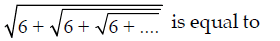(A) 2
(B) 5
(C) 4
(D) 3

6. The sum of the squares to two natural consecutive odd numbers is 394. The sum of the numbers is
(A) 24
(B) 32
(C) 40
(D) 28

7. When (67th + 67) is divided by 68, the remainder is
(A) 1
(B) 63
(C) 66
(D) 67

8. A can do a piece of work in 24 days, B in 52 days and C in 64 days. All being to do it together, but A leaves after 6 days and B leaves 6 days before the completion of the work. How many days did the work last?
(A) 15
(B) 20
(C) 18
(D) 30

9. The square root of (0.75)3/1 – 0.75 + [0.75 + (0.75)2 + 1] is
(A) 1
(B) 2
(C) 3
(D) 4

10. Given that Given that √4096+ √40.96+ √0.004096 is
(A) 70.4
(B) 70.464
(C) 71.104
(D) 71.4

11. The least positive integer that should be subtracted from 3011 x 3012 so that the different is a perfect square is
(A) 3009
(B) 2010
(C) 3011
(D) 3012

12. P, Q, R are employed to do a work for Rs. 5750. P and Q together finished 19/23 of work AND Q and R together finished 8/23 of work. Wage of Q, in rupees, is
(A) 2850
(B) 3750
(C) 2750
(D) 1000

13. While selling, a businessman allows 40% discount on the marked price and there is a loss of 30%. If it is sold at the marked price, profit percent will be
(A) 10%
(B) 20%
(C) 16 2/3%
(D) 16 1/3 %

14. A cistern has 3 pipes A, B and C. A and B call fill it in 3 and hours respectively, and C can empty it in 1 hour. If the pipes are opened at 3 p.m., 4 p.m. and 5 p.m. respectively on the same day, the cistern will be empty at
(A) 7 : 12 p.m.
(B) 7 : 15 p.m.
(C) 7 : 10 p.m.
(D) 7 : 18 p.m.

15. If A works alone, he would take 4 days more to complete the job than if both A and B worked together. If B worked alone, he would take 16 days more to complete the job than if A and B work together. How many days would they take to complete the work if both of them worked together?
(A) 10 days
(B) 12 days
(C) 6 days
(D) 8 days

16. 250 men can finish a work in 20 days working 5 hours a day. To finish the work within 10 days working 8 hours a day, the minimum number of men required is
(A) 310
(B) 300
(C) 313
(D) 312

17. 2 men and 5 women can be a work in 12 days. 5 men and 2 women can do that work in 9 days. Only 3 women can finish the same work in
(A) 36 days
(B) 21 days
(C) 30 days
(D) 42 days

18. By selling an article at 3/4 th of the marked price, there is a gain of 25%. The ratio of the marked price and the cost price is
(A) 5 : 3
(B) 3 : 5
(C) 3 : 4
(D) 4 : 3

19. A and B earn in the ratio 2 : 1. They spend in the ratio 5 : 3 and save in the ratio 4 : 1. If the total monthly savings of both A and B are Rs. 5,000, the monthly income of B is
(A) Rs. 7,000
(B) Rs. 14,000
(C) Rs. 5,000
(D) Rs. 10,000

20. The ratio of the sum of two numbers and their difference is 5 : 1. The ratio of the greater number to the smaller number is
(A) 2 : 3
(B) 3 : 2
(C) 5 : 1
(D) 1 : 5

21. Successive discounts of 10%, 20% will be equivalent to a single discount is
(A) 36%
(B) 64%
(C) 80%
(D) 56%

22. A retailer offers the following discount scheme for buyers on an article.
I. Two successive discounts of 10%.
II. A discount of 12% follows by a discount of 8%.
III. Successive discounts of 15% and 5%.
IV. A discount of 20%.
The selling price will be minimum under the scheme.
(A) I
(B) II
(C) III
(D) IV

23. A mixture contains 80% acid and rest water. Part of the mixture that should be removed and replaced by the same amount of water to make the ratio of acid and water 4 : 3 is
(A) 1/3rd
(B) 3/7 th
(C) 2/3 rd
(D) 2/7 th

24. An employer reduces the number of his employees in the ration 9 : 8 and increases their wages in the ration 14 : 15. If the original wage was Rs, 18,900, find the ratio in which the wage bill is decreased.
(A) 20 : 21
(B) 21 : 20
(C) 20 : 19
(D) 19 : 21

25. The batting average for 40 innings of a cricketer is 50 runs. His highest score by 172 runs. If these two innings are excluded, the average of the remaining 38 innings is 48 runs. The highest score of the player is
(A) 165
(B) 170
(C) 172
(D) 174

26. A and B are two alloys of gold and copper prepared by mixing metals in the ratio 7:2 and 7:11 respectively. If equal quantities of the alloys are melted to form a third alloy C, the ratio of gold and copper in C will be
(A) 5 : 7
(B) 5 : 9
(C) 7 : 5
(D) 9 : 5

27. In a laboratory, two bottles contain mixture of acid and water in the ratio 2 : 5 in the first bottle and 7 : 3 in the second. The ratio in which the contents of these two bottles be mixed such that the new mixture has acid and water in the ratio 2 : 3 is
(A) 4 : 15
(B) 9 : 8
(C) 21 : 8
(D) 1 : 2

28. The average the three numbers is 154. The first number is twice the second and the second number is twice the third. The first number is
(A) 264
(B) 132
(C) 88
(D) 66

29. The average salary of all the staff in an office of a corporate house is Rs. 5,000. The average salary of the officers is Rs. 14,000 and that of the rest is Rs. 4,000. If the total number of staff is 500, the number of officers is
(A) 10
(B) 15
(C) 25
(D) 50

30. The average marks of 40 students in an English exam is 72. Later it is found that three marks 64, 62 and 84 were wrongly entered as 68, 65 and 73. The average after mistakes were rectified is
(A) 70
(B) 72
(C) 71.9
(D) 72.1

31. Of three numbers, the second is thrice the first and the third number is three-fourth of the first. If the average of the three numbers is 114, the largest number is
(A) 72
(B) 216
(C) 354
(D) 726

32. A car covers 1/5 of the distance from A to B at the speed at 8 km/hr, 1/10 of the distance at 25 km per hour and the remaining at the speed of 20 km per hour. Find the average speed of the whole journey.
(A) 12.625 km/hr
(B) 13.625 km/hr
(C) 14.625 km/hr
(D) 15.625 km/hr

33. A jar contains 10 red marbles and 30 green ones. How many red marbles must be added to the jar so that 60% of the marbles will be red?
(A) 25
(B) 30
(C) 35
(D) 40

34. If a number multiplied by 25% of itself gives a number which is 200% more than the number, then the number is
(A) 12
(B) 16
(C) 20
(D) 24

35. The value on an article depreciates every year at the rate of 10% of its value. If the present value of the article is Rs. 729, then its worth 3 years ago was
(A) Rs. 1250
(B) Rs. 1000
(C) Rs. 1125
(D) Rs. 1200

36. The price of onions has been increased by 50%. In order to keep the expenditure on onions the same the percentage of reduction in consumption has to be
(A) 50%
(B) 33 1/3%
(C) 33%
(D) 30%

37. Walking at 3 km per hour, Pintu reaches his school 5 minutes late. If he walks at 4 km per hour he will be 5 minutes late. If he walks at 4 km per hour he will be 5 minutes early. The distance of Pintu’s school from his house is
(A) 2 ½ km
(B) 2 km
(C) 2 ½ km
(D) 5 km

38. Nitin bought some organs at Rs. 40 a dozen and an equal number at Rs. 30 a dozen. He sold them at Rs. 45 a dozen and made a profit of Rs. 480. The number of organs, he bought, was
(A) 48
(B) 60
(C) 72
(D) 84

39. A man buys two chairs for a total cost of Rs. 900. By selling one for 4/5 of its cost and the other for 4/5 or its cost, he makes a profit of Rs. 90 on the whole transaction. The cost of the lower priced chair is
(A) Rs. 360
(B) Rs. 400
(C) Rs. 420
(D) Rs. 300

40. By selling 100 oranges, a vendor gains the selling price of 20 oranges. His gain percent is
(A) 20
(B) 25
(C) 30
(D) 32

41. 60% of the cost price of an article is equal to 50% of its selling price. Then the percentage of profit or loss on the cost price is
(A) 20% loss
(B) 16 2/3 %
(C) 20% profit
(D) 10% loss

42. Maninder bought two horses at Rs. 40,000 each. He sold one horse at 15% gain, but had to sell the second horse at a loss. If he had suffered a loss of Rs. 3,600 on the whole transaction, then the selling price of the second horse is
(A) Rs. 30,000
(B) Rs. 30,200
(C) Rs. 30,300
(D) Rs. 30,400

43. A fruit-seller buys x guava for Rs. y and sells y guavas for Rs. x. If x > y, the he made
(A) x2 – y2/xy % loss
(B) x2 – y2/xy % gain
(C) x2 – y2/y2 % loss
(D) x2 – y2/y2 % gain

44. If the simple interest on Rs. x at a rate of a% for m years is same as that on Rs y at a rate of a2% for m2 years, then x : y is equal to
(A) m : a
(B) am : 1
(C) 1/m : 1/a
(D) 1/am : 1

45. A took two loans altogether for Rs. 1200 from B an C. B claimed 14% simple interest per annum, while C claimed 15% per annum. The total interest paid by A in one year was Rs. 172. Then, A borrowed
(A) Rs. 800 from C
(B) Rs. 625 from C
(C) Rs. 400 from B
(D) Rs. 800 from B

46. If a regular polygon has each of its angles equal to 3/5 times of two right angles, then the number of sides is
(A) 3
(B) 5
(C) 6
(D) 8

47. A square is of area 200 sq. m. A new square is formed in such a way that the length of its diagonal of the given square. Then the area of the new square formed is
(A) 200√2 sq. m.
(B) 400√2 sq. m.
(C) 400 sq. m.
(D) 800 sq. m.

48. The heights of a cone, cylinder and hemisphere and equal. If their radii are in the ratio 2:3:1, then the ratio of the their volumes is
(A) 2 : 9 : 2
(B) 4 : 9 : 1
(C) 4 : 27 : 2
(D) 2 : 3 : 1

49. A motor-boat can travel at 10 km/hour in still water. It travelled 91 km downstream in a river and then returned to the same place, taking altogether 20 hours. Find the rate of flow of river.
(A) 3 km/hour
(B) 4 km/hour
(C) 2 km/hour
(D) 5 km/hour

50. A man driving at 3/4th of his original speed reaches his destination 20 minutes later than the usual time. Then the usual time is
(A) 45 minutes
(B) 60 minutes
(C) 48 minutes
(D) 120 minutes

51. A motor boat, travelling at the same speed, can cover 25 km in 8 hours. At the same speed, it can travel 35 km upstream and 52 km downstream in 11 hours. The speed of the steam is
(A) 2 km/hr
(B) 2 km/hr
(C) 4 km/hr
(D) 5 km/hr

52. If a sum of money placed at compound interest, compound annually, doubles itself in 5 years, then the same amount of money will be 8 times of itself in
(A) 25 years
(B) 20 years
(C) 15 years
(D) 10 years

53. A person has left an amount of Rs. 1,20,000 to be divided between his two songs ages 14 years and 12 years such that they get equal amounts when each attains 18 years of age. If the amount gets a simple interest of 5% per annum, the younger son’s share at percent is
(A) Rs. 48,800
(B) Rs. 57,600
(C) Rs. 62,400
(D) Rs. 84,400

54. If a2 + b2 + c2 = 2(a – b – c) – 3, then the value of (a – b + c) is
(A) -1
(B) 3
(C) 1
(D) -2

55. If x2 + 3x + 1 = 0, then the value of x3 + 1/x3 is
(A) -18
(B) 18
(C) 36
(D) -36

56. If xa. xb. xc = 1 then the value of a3 + b3 + c3 is
(A) 9
(B) abc
(C) a + b + c
(D) 3abc

57. Base of a right pyramid is a square, length of diagonal of the base is 24√2 m. If the volume of the pyramid is 1728 cu. m. its height is
(A) 7 m
(B) 8 m
(C) 9 m
(D) 10 m

58. The height of a right circular cone and the radius of its circular base are respectively 9 cm and 3 cm. The cone is cut by a plane parallel to its base so as to divide it into two parts. The volume of the frustum (i.e., the lover part) of the cone is 44 cubic cm. The radius of the upper circular surface of the frustum (taking π = 22/7) is
(A) 3√12 cm
(B) 3√13 cm
(C) 3√6 cm
(D) 3√20 cm

59. The ratio of radii of two right circular cylinders is 2 : 3 and their heights are in the ratio 5 : 4. The ratio of their curved surface area is
(A) 5 : 6
(B) 3 : 4
(C) 4 : 5
(D) 2 : 3

60. A solid cylinder has total surface area of 462 sq. cm. Curved surface area is 1/3 red of it’s the cylinder is
(A) 530 cm3
(B) 536 cm3
(C) 539 cm3
(D) 545 cm3

61. A cylinder and a cone have equal radii of their bases and equal heights. If their curved surface areas are in the ratio 8 : 5, the ratio of their radius and height is
(A) 1 : 2
(B) 1 : 3
(C) 2 : 3
(D) 3 : 4

62. A solid is hemispherical at the bottom and conical above. If the surface areas of the two parts are equal, then the ratio of radius and height of its conical part is
(A) 1 : 3
(B) 1 : 1
(C) √3:1
(D) 1: √3

63. Base of a right prism is an equilateral triangle of side 6 cm. If the volume of the prism is 108√3 cc. its height is
(A) 9 cm
(B) 10 cm
(C) 11 cm
(D) 12 cm

64. If a + 1/a + 2 = 0, then the value of a37 – 1/a100 is
(A) 0
(B) -2
(C) 1
(D) 2

65. The value of k for which the graph of (k – 1) x + y – 2 = 0 and (2 – k) x – 3y + 1) = 0 are parallel is
(A) 1.2
(B) -1/2
(C) 2
(D) -2

66. The length of a shadow of a vertical tower is 1/√3 times its height. The angle of elevation of the Sun is
(A) 30°
(B) 45 °
(C) 60 °
(D) 90 °

67. The graph of x + 2y = 3 and 3x – 2y = 1 meet the Y-axis at two points having distance
(A) 8/3 units
(B) 4/3 unit
(C) 1 units
(D) 2 units

68. If x + 1/16x = 1 , then the value of 64×3+ 1/64×3 is
(A) 4
(B) 52
(C) 64
(D) 76

69. If a, b, c are three non-zero real numbers such that a + b + c = 0, and b2 ca, then the value of a2 + b2 + c2/b2-ca is
(A) 3
(B) 2
(C) 0
(D) 1

70. If a2+ a2 b2 + b4 =8 and a2 + ab + b2 = 4, then the value of ab is
(A) -1
(B) 0
(C) 2
(D) 1

71. If a = 25, b = 15, c = -10, then the value of a3+b3+c3-3abc/(a-b)2+(b-c)2+(c-a)2 is
(A) 30
(B) 15
(C) -30
(D) -15

72. A, B, C are three points on a circle. The tangent at A meets BC produced at T, BTA = 40°, CAT = 44°. The angle subtended by BC at the centre of the circle is
(A) 84°
(B) 92°
(C) 96°
(D) 104°

73. If the length of a chord of a circle at a distance of 12 cm from the centre is 10 cm, then the diameter of the circle is
(A) 13 cm
(B) 15 cm
(C) 26 cm
(D) 30 cm

74. In ABC, P and Q are the middle points of the sides AB and AC respectively. R is a point on the segment PQ such that PR : RQ = 1 : 2. If PR = 2cm. then BC =
(A) 4 cm
(B) 2 cm
(C) 12 cm
(D) 6 cm

75. If O is the circumventer of ABC and OBC = 35°, then the BAC is equal to
(A) 55°
(B) 110°
(C) 70°
(D) 35°

76. If I is the incentre of ABC and BIC = 135°, then ABC is
(A) acute angled
(B) equilateral
(C) right angled
(D) obtuse angled

77. If sin2 α + sin2 β, then the value of cos α + β/2 is
(A) 1
(B) -1
(C) 0
(D) 0.5

78. The value of cot π/20 cot 3π/20 cot 5π/20 cot 7π/20 cot 9π/20 is
(A) -1
(B) 1/2
(C) 0
(D) 1

79. If sin Ө +cos Ө= 17/13, 0 < Ө < 90°, then the value of sin Ө – cos Ө is
(A) 5/17
(B) 3/19
(C) 7/10
(D) 7/13

80. If tan Ө .tan2 Ө = 1, then the value of sin22 Ө + tan22 Ө is equal to
(A) 3/4
(B) 10/3
(C) 3 ¾
(D) 3

Directions (81-85): The following line diagram represents the yearly sales figure of a company in the years 2001 – 1010. Examine the diagram and answer the question 81 to 85.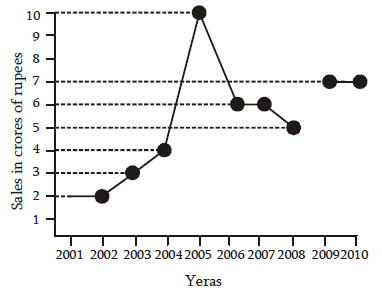81. By what percent did the sales in 2008 decrease in comparison to the sales in 2006?
(A) 20
(B) 18
(C) 16 2/3
(D) 15 2/3

82. The ratio of sales in 2002 to that in 2007 is
(A) 2 : 3
(B) 1 : 3
(C) 1 : 1
(D) 3 : 2

83. Average sale (in crore of Rs.) of the company during the period 2003 – 2007 is
(A) 5.8
(B) 5
(C) 6
(D) 5.5

84. The percentage increase in sales in the year 2005 with respect to the previous year is
(A) 80
(B) 100
(C) 120
(D) 150

85. Total sales (in crore of Rs.) from 2005 to 2008 is
(A) 17
(B) 27
(C) 22
(D) 31

Directions (86-90: The following table shows the number of students of 7 college participating in extracurricular activities: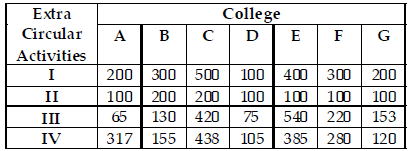86. The different of the range of number of students in activity IV and the average of number of students in activity III per college is
(A) 111
(B) 153
(C) 104
(D) 217

87. Percentage of the number of students in activity II to that of IV is
(A) 37
(B) 42
(C) 48
(D) 50

88. The college in which minimum number of students particular in extracurricular activities is
(A) 540
(B) 229
(C) 153
(D) 75

89. The college in which minimum number of students participate in extracurricular activities is
(A) D
(B) G
(C) F
(D) A

90. The ratio of total number of students in II and I is
(A) 1 : 2
(B) 9 : 20
(C) 19 : 7
(D) 21 : 10

Directions (91 – 95): The pie-chart provided below gives the distribution of land (in a village) under various food crops. Study the pie-chart carefully and answer the questions from 91 to 95.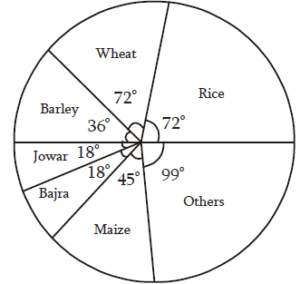91. If the total area under bajra was three hundred acres, then the total area (in hundred acres) under rice and barley together is
(A) 18
(B) 12
(C) 15
(D) 20

92. The combination of three crops which contribute to more than 50% of the total area under the food crops is
(A) Wheat, rice and maize
(B) wheat, rice and jowar
(C) wheat, rice and bajra
(D) rice, barley and maize

93. The ratio of the land used for rice and barley is
(A) 3 : 1
(B) 1 : 2
(C) 2 : 1
(D) 3 : 2

94. If 10% of the land reserved for rice be distributed to wheat and barley in the ratio 2 : 1, then the angle corresponding to wheat in the new pie-chart will be
(A) 38.4°
(B) 76.8°
(C) 75.6°
(D) 45.5°

95. If the production of rice is 5 times that of jowar and the production of jowar is 2 times that of bazra, then the ratio between the yield per acre of rice and bazra is
(A) 5 : 1
(B) 3 : 1
(C) 4 : 1
(D) 6 : 1

Directions (96 – 100): The bar graph provided below represents the production of rice and wheat in different states of a country in the certain year. Answer questions 96 to 100 based on the bar graph.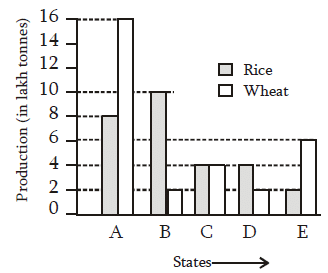96. The total production of rice and wheat in all the mentioned states in minimum in the state
(A) B
(B) C
(C) D
(D) E

97. The ratio of total production of rice in the mentioned states to that of wheat in those states, is
(A) 15 : 16
(B) 12 : 13
(C) 13 : 14
(D) 14 : 15

98. The difference between the production in rice and wheat is maximum in
(A) A only
(B) All of A, B and E
(C) B and E both
(D) A and B both

99. The state which is the largest producer of rice is
(A) A
(B) B
(C) C
(D) D

100. The average of production of rice in the mentioned states (in lakh tonnes) is
(A) 5 : 5
(B) 5 : 6
(C) 5 : 7
(D) 5 : 8

1. (D)
2. (C)
3. (C)
4. (C)
5. (D)
6. (D)
7. (C)
8. (*)
9. (B)
10. (B)
11. (C)
12. (D)
13. (C)
14. (A)
15. (D)
16. (C)
17. (A)
18. (A)
19. (A)
20. (B)
21. (B)
22. (D)
23. (D)
24. (B)
25. (D)
26. (C)
27. (C)
28. (A)
29. (D)
30. (D)
31. (B)
32. (D)
33. (C)
34. (A)
35. (B)
36. (B)
37. (B)
38. (A)
39. (D)
40. (B)
41. (C)
42. (D)
43. (D)
44. (B)
45. (D)
46. (B)
47. (C)
48. (C)
49. (A)
50. (B)
51. (C)
52. (C)
53. (B)
54. (C)
55. (A)
56. (D)
57. (C)
58. (B)
59. (A)
60. (C)
61. (D)
62. (D)
63. (D)
64. (B)
65. (A)
66. (C)
67. (D)
68. (B)
69. (B)
70. (D)
71. (D)
72. (D)
73. (C)
74. (C)
75. (A)
76. (C)
77. (C)
78. (D)
79. (D)
80. (C)
81. (C)
82. (C)
83. (A)
84. (D)
85. (B)
86. (C)
87. (D)
88. (C)
89. (A)
90. (B)
91. (A)
92. (A)
93. (C)
94. (B)
95. (*)
96. (C)
97. (D)
98. (D)
99. (B)
100. (B)